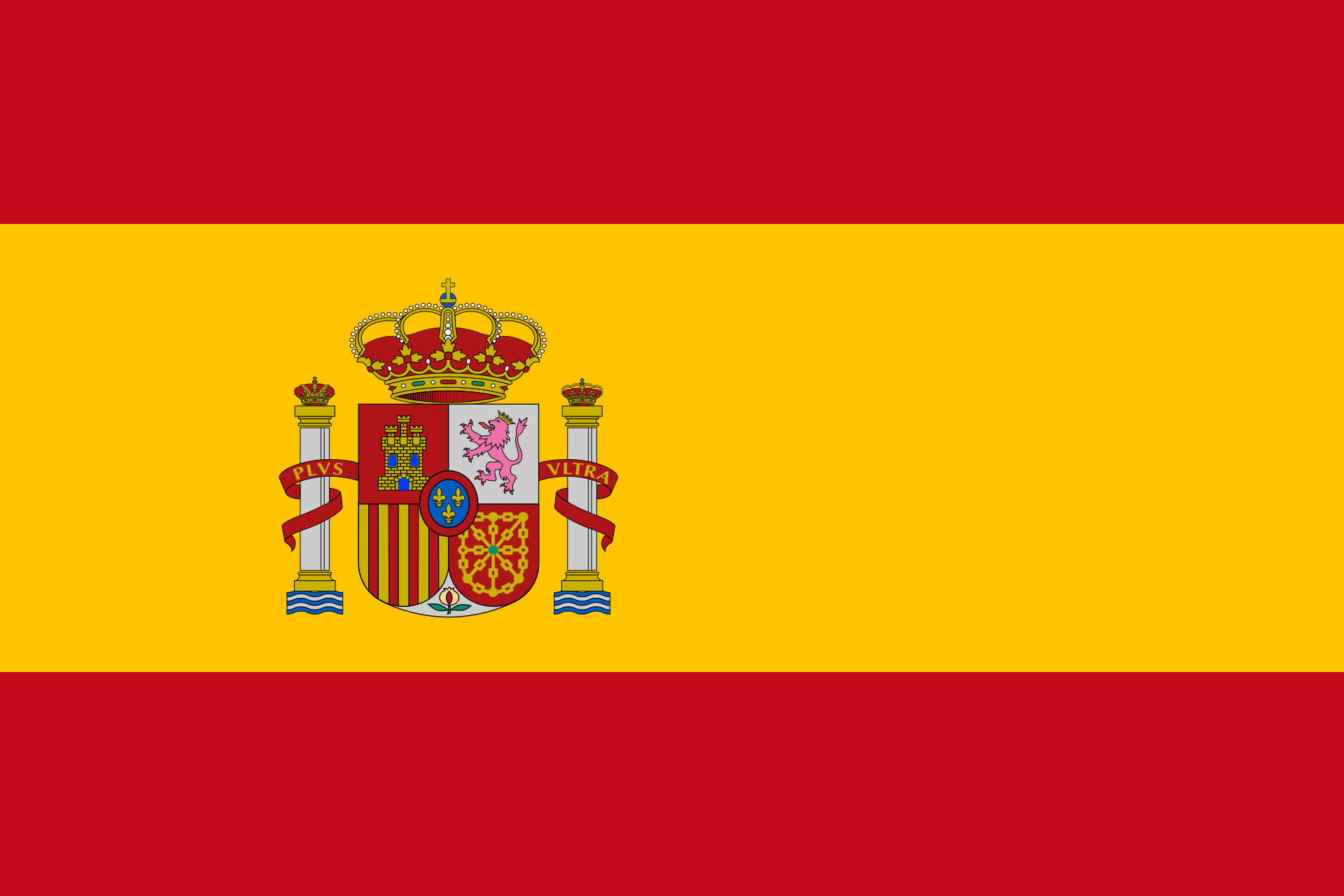Converting quadratic functions Enter your quadratic function here. Instead of x▓, you can also write x^2. Get the following form: Vertex form Normal form Factorized form
 Get a quadratic function from its roots Enter the roots and an additional point on the Graph. Mathepower finds the function and sketches the parabola. Roots at and Further point on the Graph: P(|)
 Calculate a quadratic function given the vertex point Enter the vertex point and another point on the graph. Vertex point: (|) Further point: (|)
 Computing a quadratic function out of three points Enter three points. Mathepower calculates the quadratic function whose graph goes through those points. Point A(|) Point B(|) Point C(|)

 Find the roots Enter the function whose roots you want to find. Hints: Enteras 3*x^2 ,as (x+1)/(x-2x^4) andas 3/5.
 Transforming functions Enter your function here. How shall your function be transformed? By in x-direction stretched shrinked By in y-direction stretched shrinked By to the the right l the left By to the up down

Find a function

Degree of the function:
 1 2 3 4 5

( The degree is the highest power of an x. )

Symmetries:
axis symmetric to the y-axis
point symmetric to the origin

y-axis intercept

Roots / Maxima / Minima /Inflection points:
at x=
at x=
at x=
at x=
at x=

Characteristic points:
at |)
at |)
at |)
at (|)
at (|)

Slope at given x-coordinates:
Slope at x=
Slope at x=
Slope at

Quadratic functions are functions of the form. This means, there is no x to a higher power than. The graph of a quadratic function is a parabola.